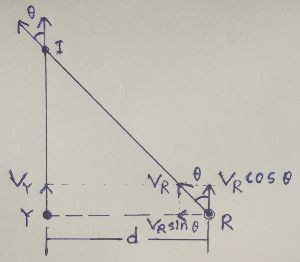# A zebra sees a lion 300 m to his right. The zebra starts running at 25 kph straight ahead to get...

## Question:

A zebra sees a lion 300 m to his right. The zebra starts running at 25 kph straight ahead to get away. At this point the lion launches himself on an intercept course at the zebra at a speed of 31 kph.

a. At what angle does the lion launch himself?

b. If both animals continue to travel straight, how long does it take the lion to catch the zebra?

## Prey Predator Interception:Let {eq}Y {/eq} and {eq}R {/eq} be two points at which there are a prey and predator present at the same time and they see each other. The prey, on seeing the predator to its right at a distance {eq}d {/eq}, starts running straight ahead with velocity {eq}V_Y {/eq} and the predator, on seeing its prey running, also begins running straight ahead at the same time, as if with calculative velocity {eq}V_R {/eq} at an angle {eq}\theta {/eq} with direction of velocity of the prey.

Then, from above conditions, we can write:

{eq}\displaystyle{V_R\, \cos\theta = V_Y} {/eq}.

Therefore, required angle between the directions of velocity of the predator and that of the prey can be given by:

{eq}\displaystyle{\theta = \cos^{-1}\left(\frac{V_Y}{V_R}\right)} {/eq}.

Time it takes the predator to intercept the prey is given by:

{eq}\displaystyle{t = \frac{d}{\sqrt{V^{2}_R - V^{2}_Y}}} {/eq}.

Distance run by the prey is given by:

{eq}\displaystyle{d_Y = \frac{d\, V_Y}{\sqrt{V^{2}_R - V^{2}_Y}}} {/eq}.

Distance run by the predator is given by:

{eq}\displaystyle{d_R = \frac{d\, V_R}{\sqrt{V^{2}_R - V^{2}_Y}}} {/eq}

Initial distance between the lion and the zebra: {eq}\color{blue}{d = 300\, \rm m} {/eq}.

Magnitude of velocity of the zebra: {eq}\color{blue}{V_Z = 25\, \rm kmph} {/eq}.

Magnitude of velocity of the lion: {eq}\color{blue}{V_L = 31\, \rm kmph} {/eq}.

#### a)

Therefore angle which the lion launches itself with the direction of the motion of the zebra is given by:

{eq}\displaystyle{\begin{align*} \color{red}{\theta} &= \color{blue}{\cos^{-1}\left(\frac{V_Z}{V_L}\right)}\\ &= \color{blue}{\cos^{-1}\left(\frac{25}{31}\right)}\\ &\approx \color{red}{36.25^\circ}\\ \end{align*}} {/eq}

Time it takes the lion to intercept the zebra is given by:

{eq}\displaystyle{\begin{align*} \color{red}{t} &= \color{blue}{\frac{d}{\sqrt{V^{2}_L - V^{2}_Z}}}\\ &= \color{blue}{\frac{300}{\sqrt{31^{2} - 25^{2}}\times \frac{5}{18}}}\, \, \, \, \, \, \, \, \, \, \left(\because 1\, \rm kmph = \frac{5}{18}\, \rm m/s\right)\\ &= \color{red}{58.92\, \rm s}\\ \end{align*}} {/eq}